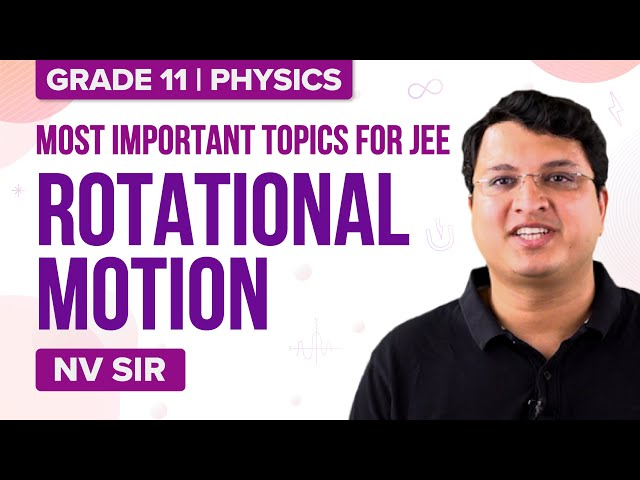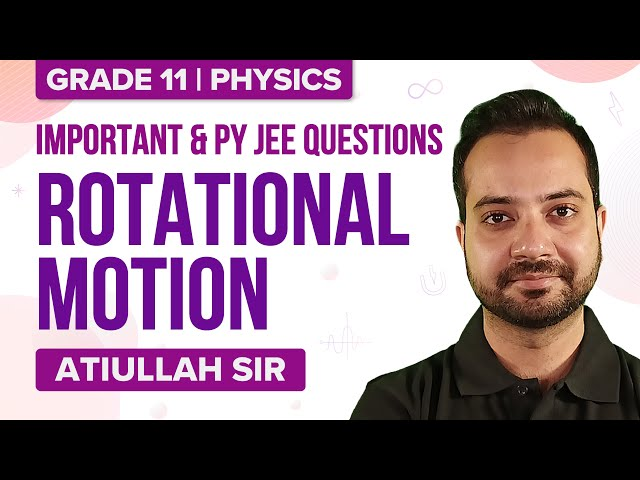Checkout JEE MAINS 2022 Question Paper Analysis : Checkout JEE MAINS 2022 Question Paper Analysis :

# JEE Main Rotational Dynamics Previous Year Questions with Solutions

In our daily life, we come across many objects that undergo rotational motion. Let us consider the example of a cylinder rolling down an inclined plane. The cylinder follows both translational motion and rotational motion. In rotational motion, the particles of the body follow a circular path along a plane that is perpendicular to the axis and its centre will lie on the same plane. Therefore, we can define rotational motion as the circular motion of an object along an axis. The axis can be either fixed or unfixed. An example of rotational motion about a fixed axis is a fan and an example of an unfixed axis is a spinning top.

As we know that in linear motion or translational motion an object is moved till it feels any external force. This external force applied changes the motion of objects. The force that is applied in rotational motion is known as torque. It is easier to open a door by pushing on the edge farthest from the hinges than by pushing in the middle. Thus, the torque is a force which is studied under rotational dynamic.

## JEE Main Previous Year Solved Questions on Rotational Dynamics

Past year’s important questions asked in IIT JEE from the topic “Rotational Dynamics” are listed below.

Q1: Two-point masses of 0.3kg and 0.7kg are fixed at the ends of a rod which is of length 1.4m and of negligible mass. The rod is set rotating about an axis perpendicular to its length with a uniform angular speed. The point on the rod through which the axis should pass in order that the work required for rotation of the rod is minimum is located at a distance of

1. 0.42 m from the mass of 0.3kg
2. 0.70 m from the mass of 0.7kg
3. 0.98m from the mass of 0.3kg
4. 0.98m from the mass of 0.7kg

Solution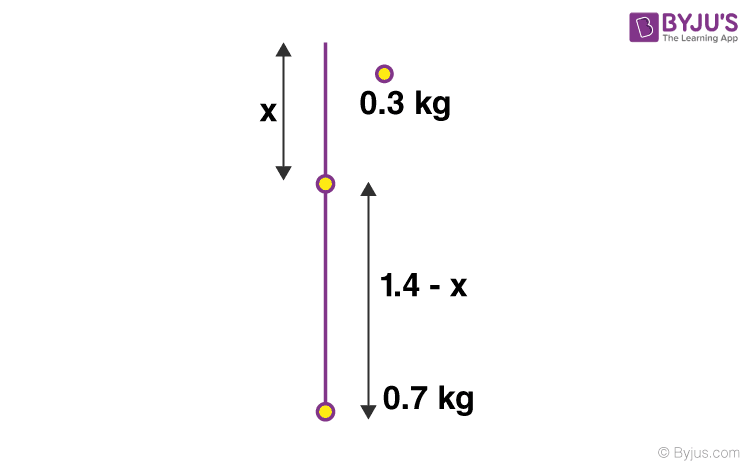The moment of inertia of the system about the axis of

rotation O is

I = I1 + I2 = (0.3)x2 + 0.7 (1.4 -x)2

I = 0.3x2+ 0.7(1.96 + x2– 2.8 x)

= x2 + 1.372 – 1.96x

The work done in rotating the rod is converted into its rotational kinetic energy.

W = ½ Iω2

= ½[x2 + 1.372 – 1.96]ω2

For the work done to be minimum

dW/dx = 0 ⇒2x – 1.96 =0

x = 1.96/2 = 0.98m

Answer: (3) 0.98m from the mass of 0.3kg

Q2: A particle undergoes uniform circular motion. About which point on the plane of the circle, will the angular momentum of the particle remain conserved?

1. centre of the circle
2. on the circumference of the circle
3. inside the circle
4. outside the circle

Solution

The force will pass through the centre of the circle. Therefore, the angular momentum will remain conserved at the centre of the circle.

Answer: (1) centre of the circle

Q3: A child is standing with folded hands at the centre of a platform rotating about its central axis. The kinetic energy of the system is K. The child now stretches his arms so that the moment of inertia of the system doubles. The kinetic energy of the system now is

1. 2K
2. K/2
3. K/4
4. 4K

Solution:

According to the conservation of angular momentum

0 = I1ω1

So we have I1 =2I

ω1 = ω0 /2

Initial kinetic energy =Iω02/2 =K

Final Kinetic energy = I1ω12/2 = 2I(ω0/2)2/2 =K/2

Q4: A particle is confined to rotate in a circular path with decreasing linear speed. Then which of the following is correct?

1. angular momentum is conserved about the centre
2. only the direction of L is conserved
3. it spirals towards the centre
4. its acceleration is towards the centre

Solution: L is not conserved in magnitude since v is changing (decreasing). It is given that a particle is confined to rotate in a circular path, it cannot have a spiral path. Since the particle has two accelerations ac and at therefore the net acceleration is not towards the centre. The direction of L remains the same even when the speed decreases.

Answer: (2) only the direction of L is conserved

Q5: A bob of mass M is suspended by a massless string of length L. The horizontal velocity V at position A is just sufficient to make it reach point B. The angle at which the speed of the bob is half of that at A satisfies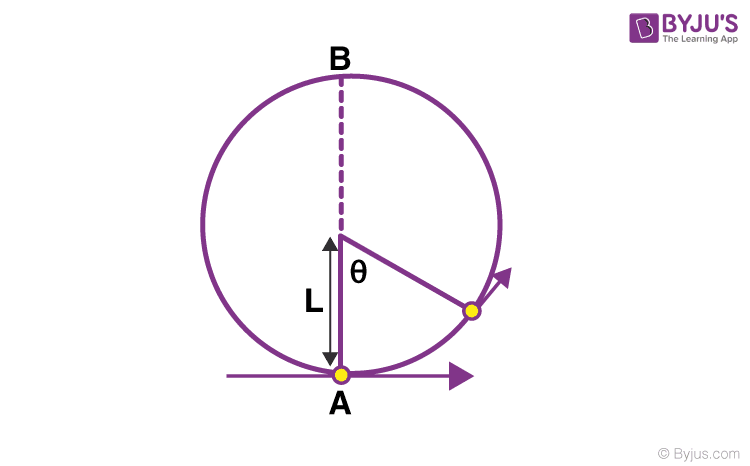1. θ= π/2
2. π/4 くθ くπ/2
3. π/2 くθ く3π/4
4. 3π/2 くθ くπ

Solution

This is the case of vertical motion when the body just

completes the circle. Here

$$\begin{array}{l}v = \sqrt{5gL}\end{array}$$

Applying energy conservation,

½ mv02 = ½ mv2 + mgl(1-cosθ)———-(1)

where v0 is the horizontal velocity at the bottom point, v is the velocity of bob where the bob inclined θ with vertical.

Also, we know the relation between the velocity at the topmost and velocity at the bottom point.

mg(2l) =½ mv02 -1/2mvtop2———-(2)

Since v0 is just sufficient

mvtop2/l = T + mg

T = 0

$$\begin{array}{l}v_{top}=\sqrt{gl}\end{array}$$

Then equation 2 becomes

$$\begin{array}{l}v_{0}=\sqrt{5gl}\end{array}$$

According to the question v= v0/2

So from equation (1)

½ m(5gl) = ½ m (5gl/4) + mgl(1-cosθ)

(20mgl – 5mgl)/8 = mgl(1-cosθ)

(1-cosθ) = 15/8

Cosθ = ⅞

Hence, 3π/2 くθ くπ

Q6: A ball of mass (m) 0.5 kg is attached to the end of a string having a length (L) 0.5 m. The ball is rotated on a horizontal circular path about a vertical axis. The maximum tension that the string can bear is 324 N. The maximum possible value of the angular velocity of ball (in radian/s) is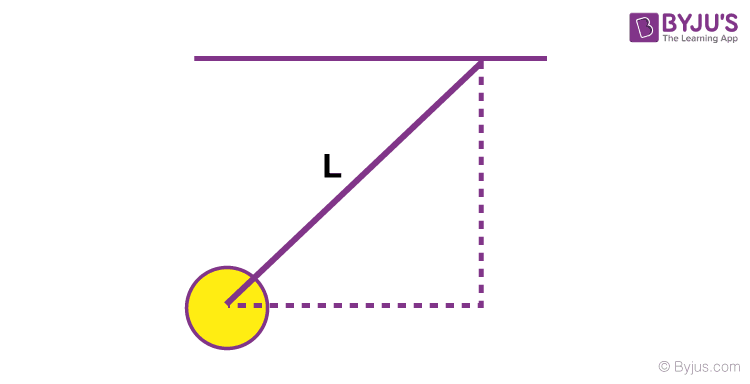(a) 9 )

(b).18

(c) 27

(d) 36

Solution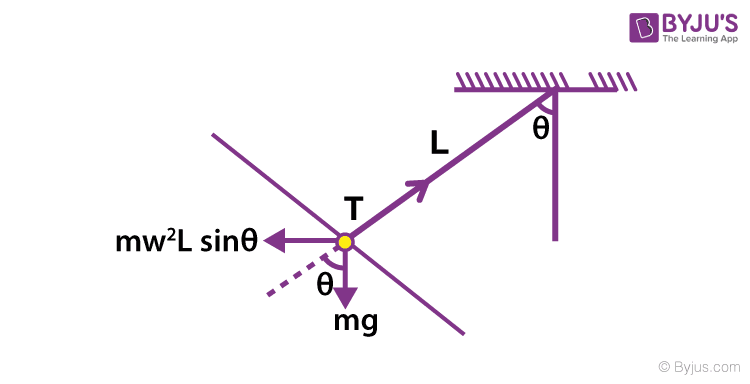2Lsinθcosθ = mgsinθ

Cos θ = g/ω2L

Sin θ = 1/ω2L

$$\begin{array}{l}sin\theta =\frac{1}{\omega ^{2}L}\sqrt{(\omega ^{2}L)^{2}-g^{2}}\end{array}$$

T=mgcosθ+ mω2Lsin2θ

T = mg(g/ω2L) + (mω22L)2((ω2L)2 -g2)

T = mg/ω2L[g2 + (ω2L)2 – g2]

T = mω2L = 324 (given)

$$\begin{array}{l}\omega =\sqrt{\frac{324}{0.5\times 0.5}}\end{array}$$

Q8: A thin ring of mass 2 kg and radius 0.5 m is rolling without slipping on a horizontal plane with velocity 1 m/s. A small ball of mass 0.1 kg, moving velocity 20 m/s in the opposite direction hits the ring at a height of 0.75 m and goes vertically up with velocity 10 m/s. Immediately after the collision.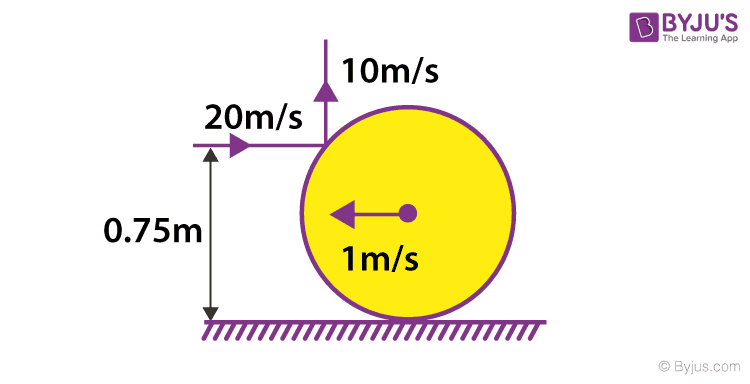(a) The ring has pure rotation about its stationary CM

(b) The ring comes to a complete stop

(c) Friction between the ring and the ground is to the left

(d) There is no friction between the ring and the ground

Solution: Let’s assume that friction between the ground and the ring gives no impulse during the collision with the ball. Using conservation of momentum along the x-axis we get that the CM of the ring will come to rest. Thus option A is correct.

Secondly, the question tells us that the ball gets a velocity in the vertical direction, hence there must be an impulse in the vertical direction. There will be horizontal and a vertical impulse on the ring at the point of contact. These will have components along the tangent of the ring, which will provide angular impulses.

Using angular impulse = change in angular momentum, we get

=2cos300(1/2) -1sin300(1/2)=2(¼)(ω2 – ω1)

note that we have assumed that the direction of angular velocities is the same before and after and since LHS of the above equation is positive ​ω2 > ω1 so the ring must be slipping to right and hence the friction will be to the left as it will be opposite to the direction of motion. Thus option C is correct

Q9: A carpet of mass M, made of inextensible material is rolled along its length in the form of a cylinder of radius R and is kept on the rough floor. The carpet starts unrolling without sliding on the floor when a negligibly small push is given to it. Calculate the horizontal velocity of the axis of the cylindrical part of the carpet when its radius reduces to R/2.

1. $$\begin{array}{l}\sqrt{\frac{14}{3}gR}\end{array}$$
2. $$\begin{array}{l}\sqrt{\frac{7}{3}gR}\end{array}$$
3. $$\begin{array}{l}\sqrt{2gR}\end{array}$$
4. $$\begin{array}{l}\sqrt{gR}\end{array}$$

Solution

The radius is reduced to R/2, the mass of the rolled carpet = (M/πR2l) x (R/2)2l =M/4

Release of potential energy = MgR- (M/4)gR/2 = ⅞MgR

The kinetic energy at this instant is given by = ½ Mv2+½Iω2 = ½ (M/4)v2+ ½(MR2/32)(2v/R)2= (3/16)Mv2

(3/16)Mv2 = ⅞MgR

v =

$$\begin{array}{l}\sqrt{\frac{14}{3}gR}\end{array}$$

$$\begin{array}{l}\sqrt{\frac{14}{3}gR}\end{array}$$

Q10: A bob of mass m attached to an inextensible string of length is suspended from a vertical support. The bob rotates in a horizontal circle with an angular speed ω rad/s about the vertical. About the point of suspension

(a) Angular momentum changes in direction but not in magnitude

(b) Angular momentum changes both in direction and magnitude

(c) Angular momentum is conserved

(d) Angular momentum changes in magnitude but not in direction.

Answer: (a) Angular momentum changes in direction but not in magnitude

Q11: A roller is made by joining together two cones at their vertices O. It is kept on two rails AB and CD which are placed asymmetrically (see figure), with its axis perpendicular to CD and its centre O at the centre of the line joining AB and CD (see figure). It is given a light push so that it starts rolling with its centre O moving parallel to CD in the direction shown. As it moves, the roller will tend to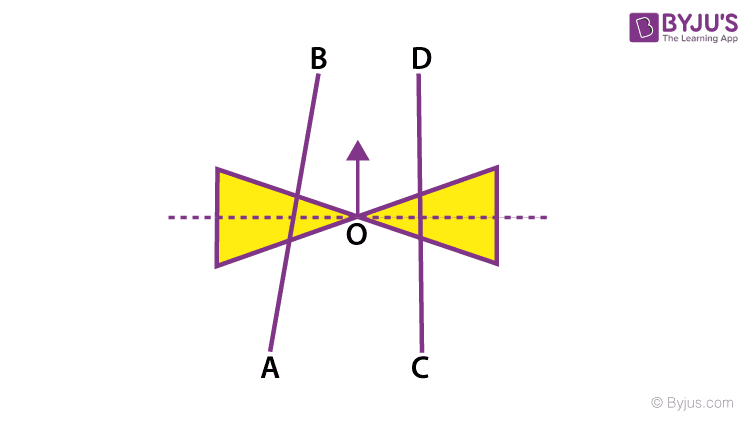(a) turn left and right alternately

(b) turn left

(c) turn right

(d) go straight

Solution:

As the cone moves forward, the line of contact in the left half tends to slip in the forward direction. As a result the radius at the left in contact decreases. The roller will move to the left.

Q12: The moment of inertia of a uniform cylinder of length and radius R about its perpendicular bisector is I. What is the ratio /R such that the moment of inertia is minimum?

(a) 1

(b)

$$\begin{array}{l}3\sqrt{2}\end{array}$$

(c)

$$\begin{array}{l}\sqrt{3}/2\end{array}$$

(d)

$$\begin{array}{l}\sqrt{3/2}\end{array}$$

Solution

Moment of Inertia I = m [I2/12 + R2/4]

Volume = πR2l

Writing Moment of inertia in terms of volume, we get

I = m/4(V/πl + I2/3)

Differentiating the above equation we get

dI/dl = m/4 (-V/πl2 + 2I/3)

For maxima and minima, dI/dl = 0

So m/4 (-V/πl2 + 2I/3) = 0

V/πl2 = 2I/3

R2/l = 2I/3 (Volume = πR2l)

l2/R2 = 3/2

$$\begin{array}{l}l/R = \sqrt{\frac{3}{2}}\end{array}$$

$$\begin{array}{l}\sqrt{3/2}\end{array}$$

Q13: From a uniform circular disc of radius R and mass 9M, a small disc of radius R/3 is removed as shown in the figure. The moment of inertia of the remaining disc about an axis perpendicular to the plane of the disc and passing through the centre of the disc is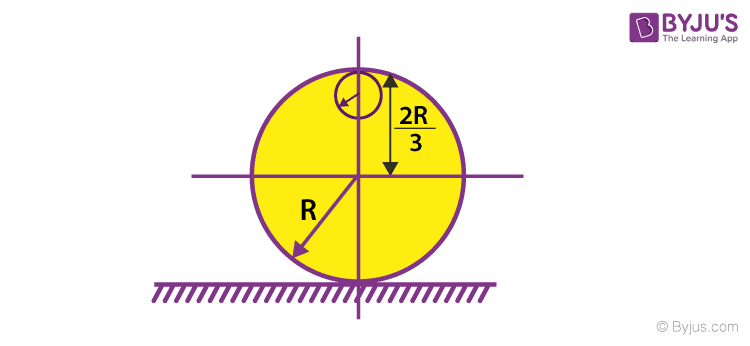(a) (40/9)MR2

(b) 10MR2

(c) (37/9)MR2

(d) 4MR2

Solution

Mass of the disc given = 9M

Mass of the removed portion = M

The moment of inertia of the complete disc about an axis passing through its centre O and perpendicular to its plane is I1= 9/2 MR2

The moment of inertia of the disc with portions removed

I2 = ½M(R/3)2 = (1/18)MR2

The moment of inertia of the remaining portion of the disc about O is

I = I1 – I2

I = 9(MR2/2) – MR2/18

I = 40 MR2/9

Q14: Seven identical circular planar disks, each of mass M and radius R are welded symmetrically as shown. The moment of inertia of the arrangement about the axis normal to the plane and passing through the point P is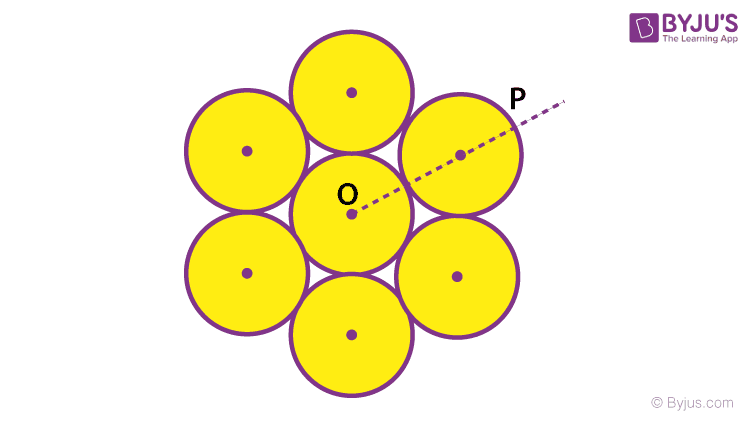1. (55/2)MR2
2. (73/2)MR2
3. (181/2)MR2
4. (19/2)MR2

Solution

I0 = Icm + md2

I0 = (7MR2/2) + 6(M x (2R)2) = 55MR2/2

Ip = I0 + md2

Ip = 55MR2/2 + 7M(3R)2 = (181/2)MR2

Q15: A pulley of radius 2 m is rotated about its axis by a force F =20t-5t2 newton (where t is measured in seconds) applied tangentially. If the moment of inertia of the pulley about its axis of rotation is 10 kgm2, the number of rotations made by the pulley before its direction of motion it reversed, is

(a) more than 6 but less than 9

(b) more than 9

(c) less than 3

(d) more than 3 but less than 6

Solution

Torque is given by τ = FR

Or α = FR/I

Given F =20t – 5t2

R = 2m,

I = 10 kgm2

α = [(20t – 5t2) x 2]/10

α = 4t – t2

$$\begin{array}{l}\omega =\int_{0}^{t}\alpha dt=2t^{2} – t^{3}/3\end{array}$$

at ω = 0 ⇒ 2t2 -t3/3 = 0

t3 = 6t2

t = 6

$$\begin{array}{l}\theta =\int_{0}^{6}\omega dt= \int_{0}^{6}(2t^{2}-t^{3}/3)dt\end{array}$$

θ = 36/2π く6

Answers: (d) more than 3 but less than 6